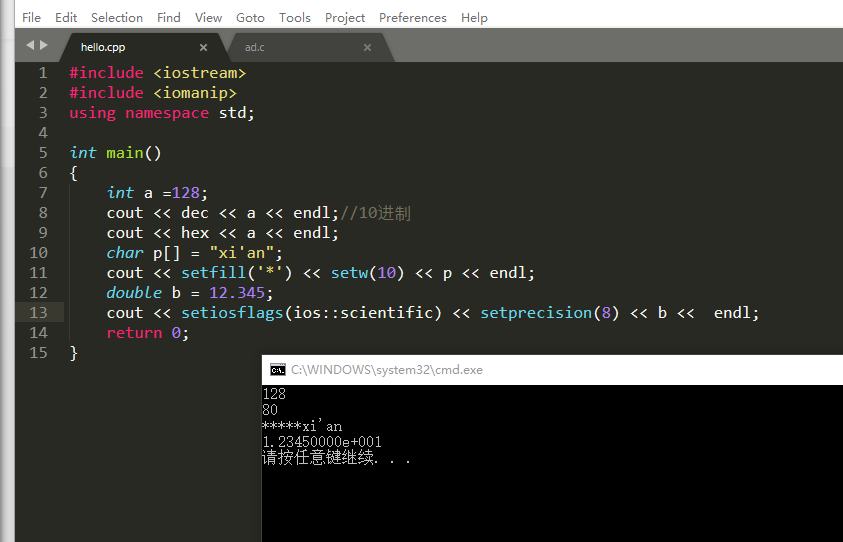# c++标准输入输出流（一）

1.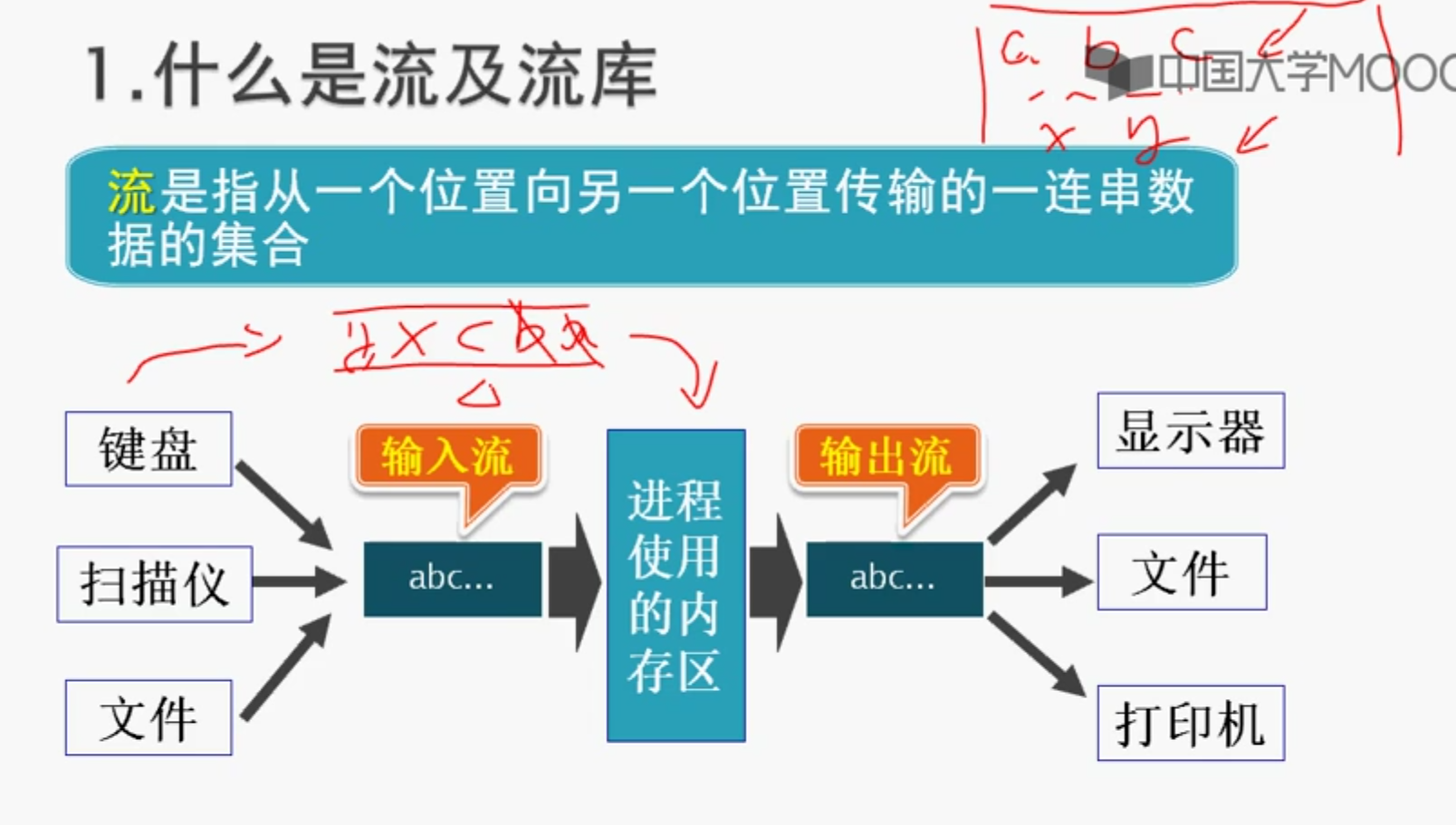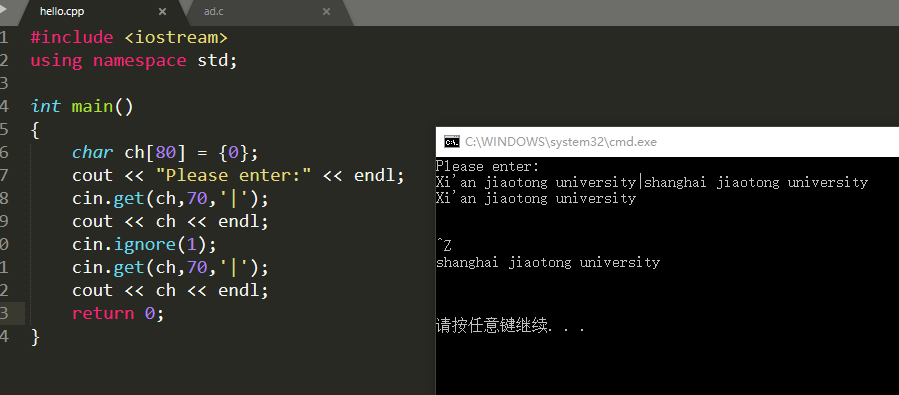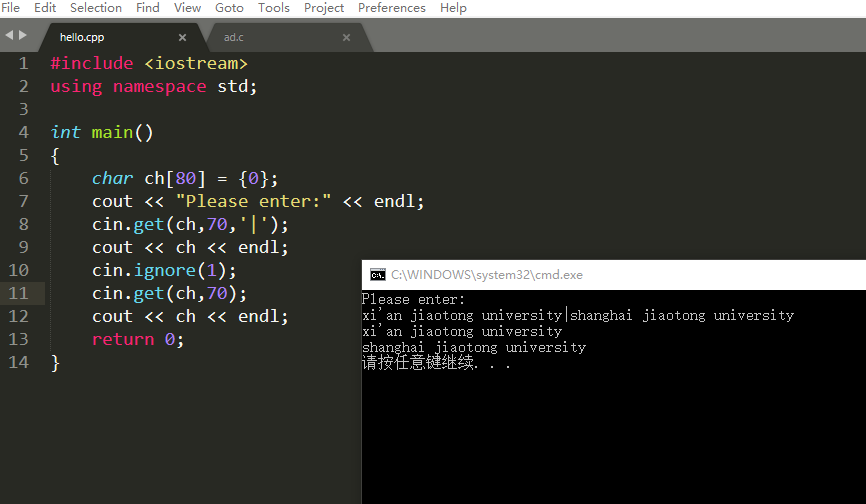2.getline
getline和get的区别是getline读取的终止字符会被舍弃。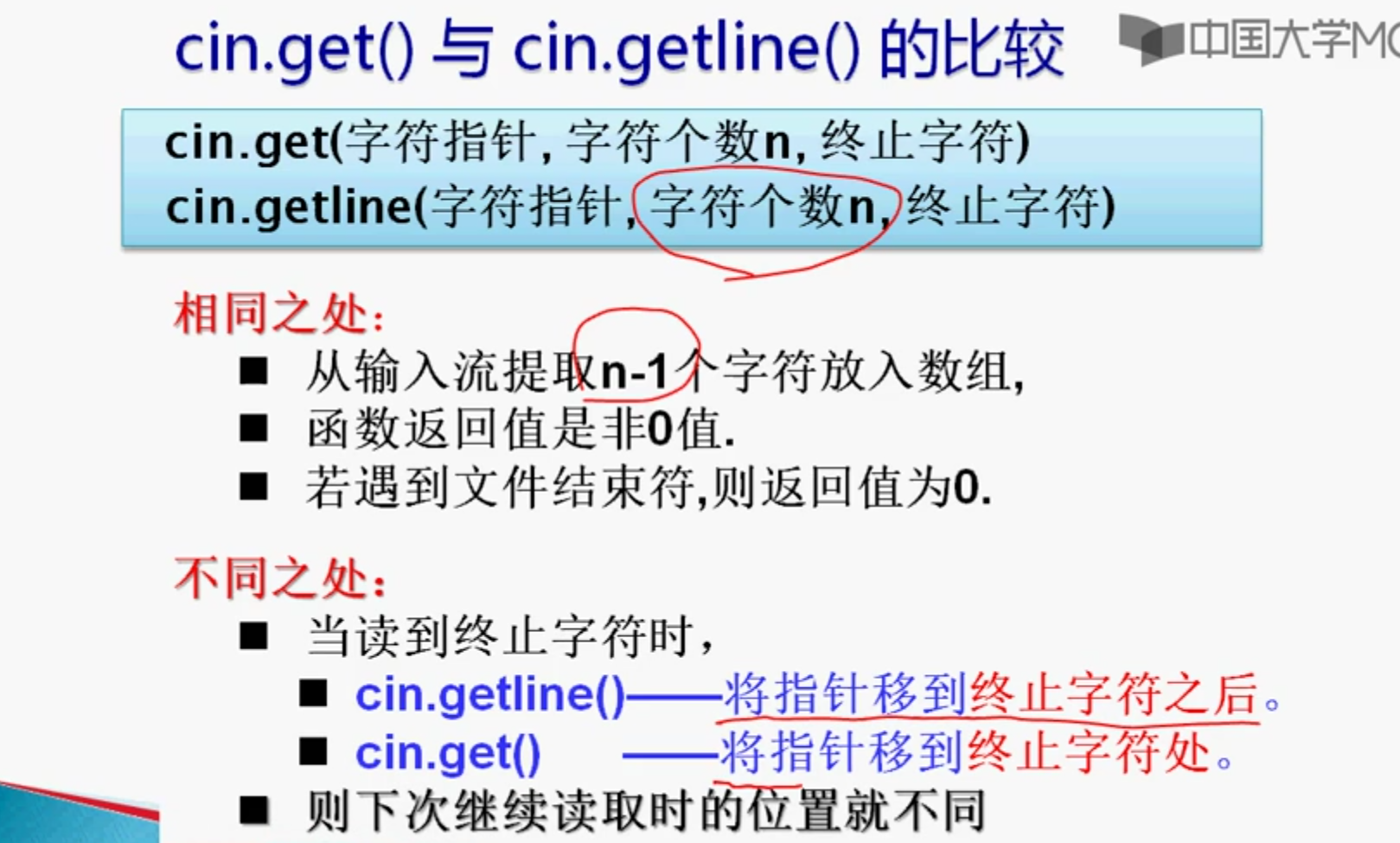3.标准输出流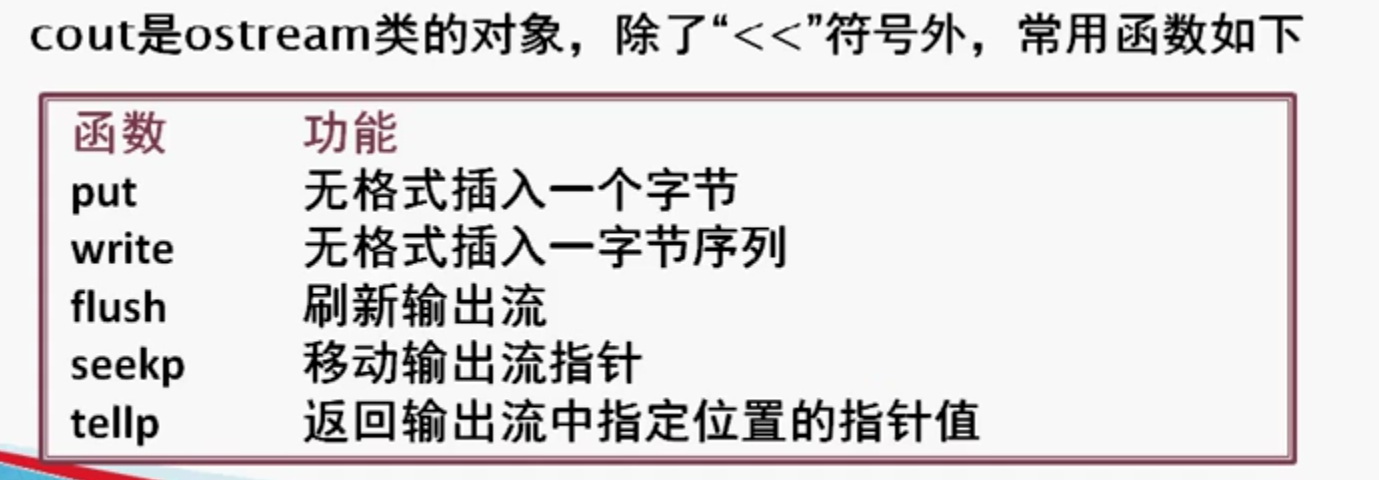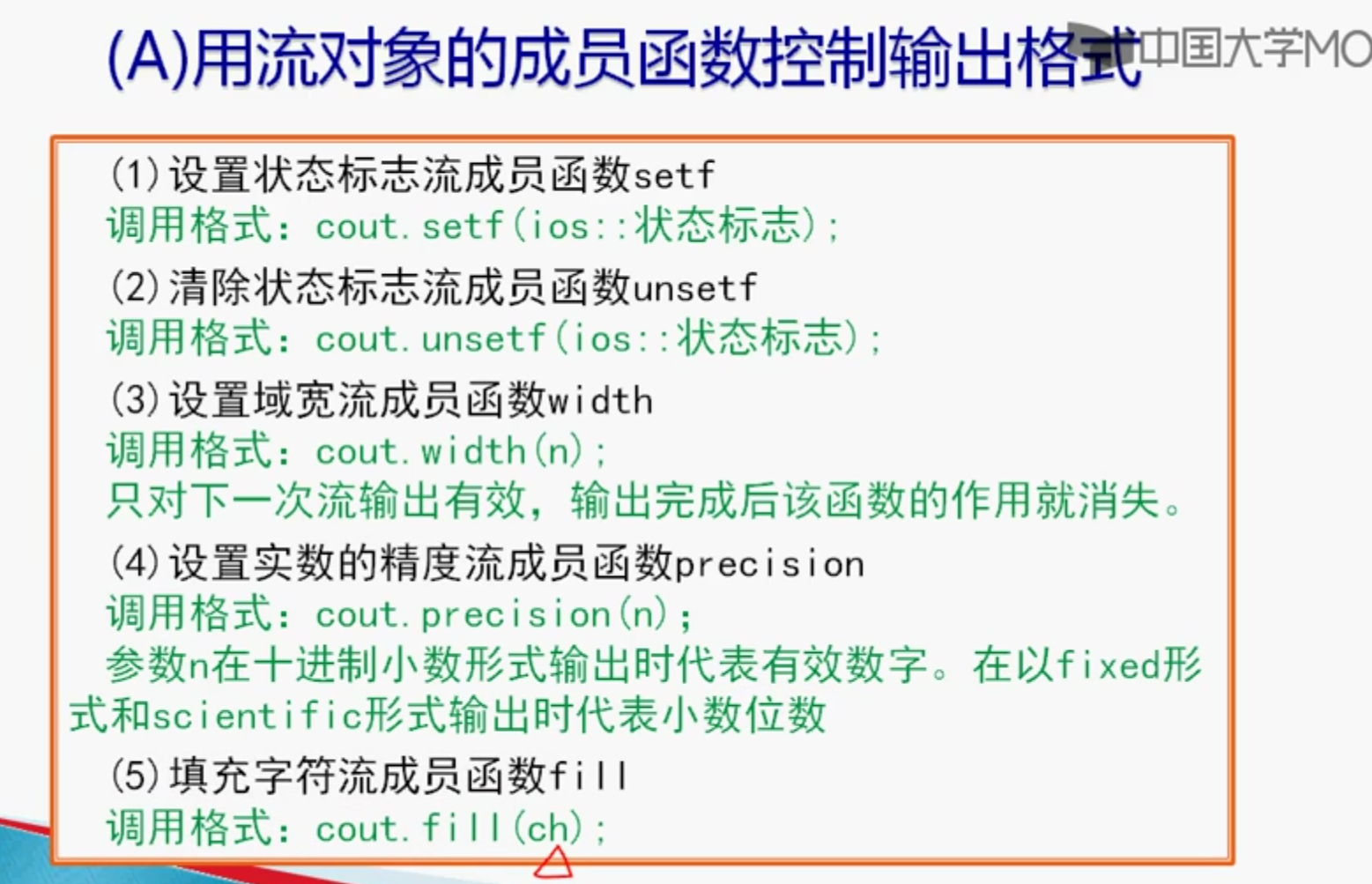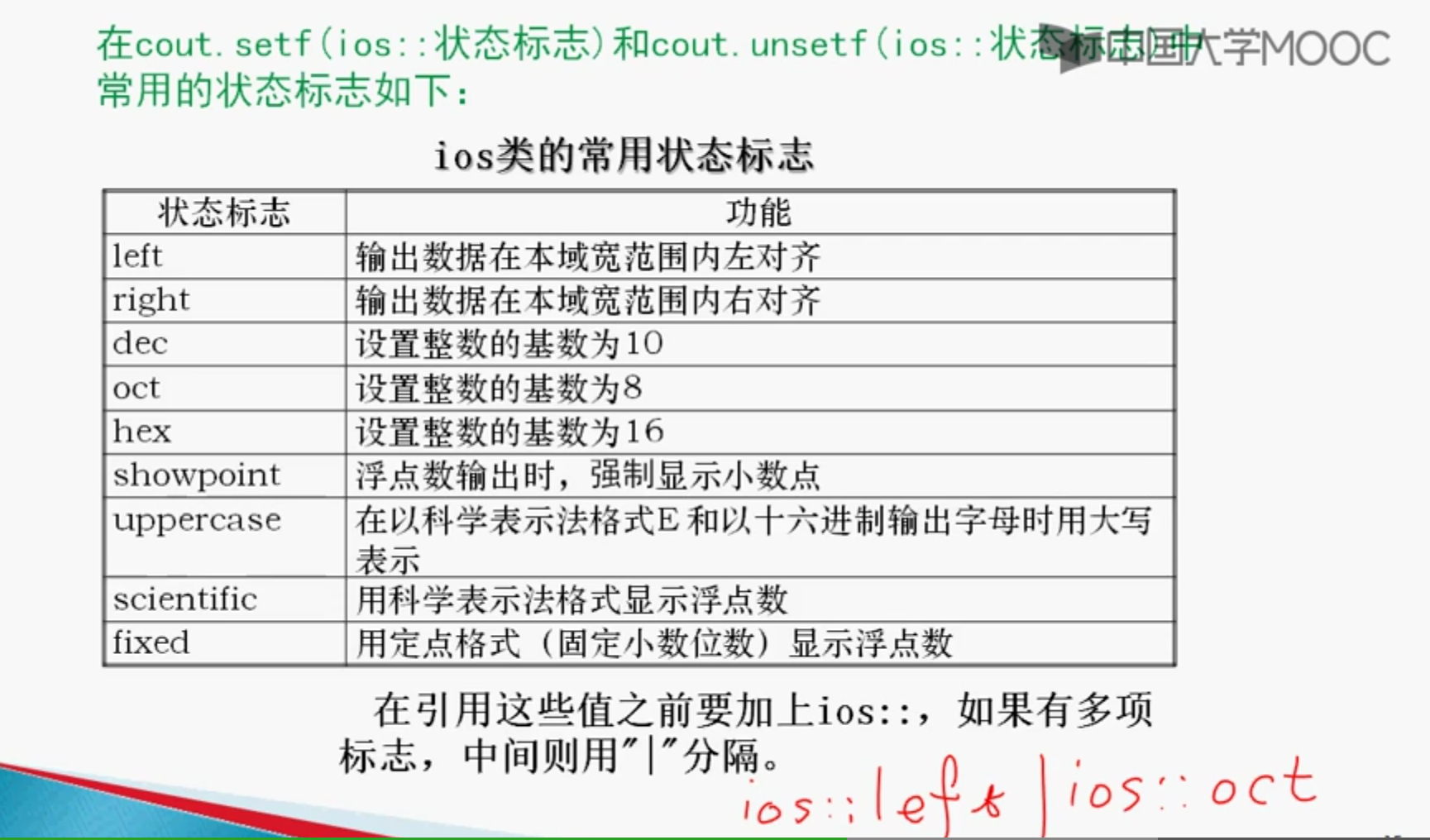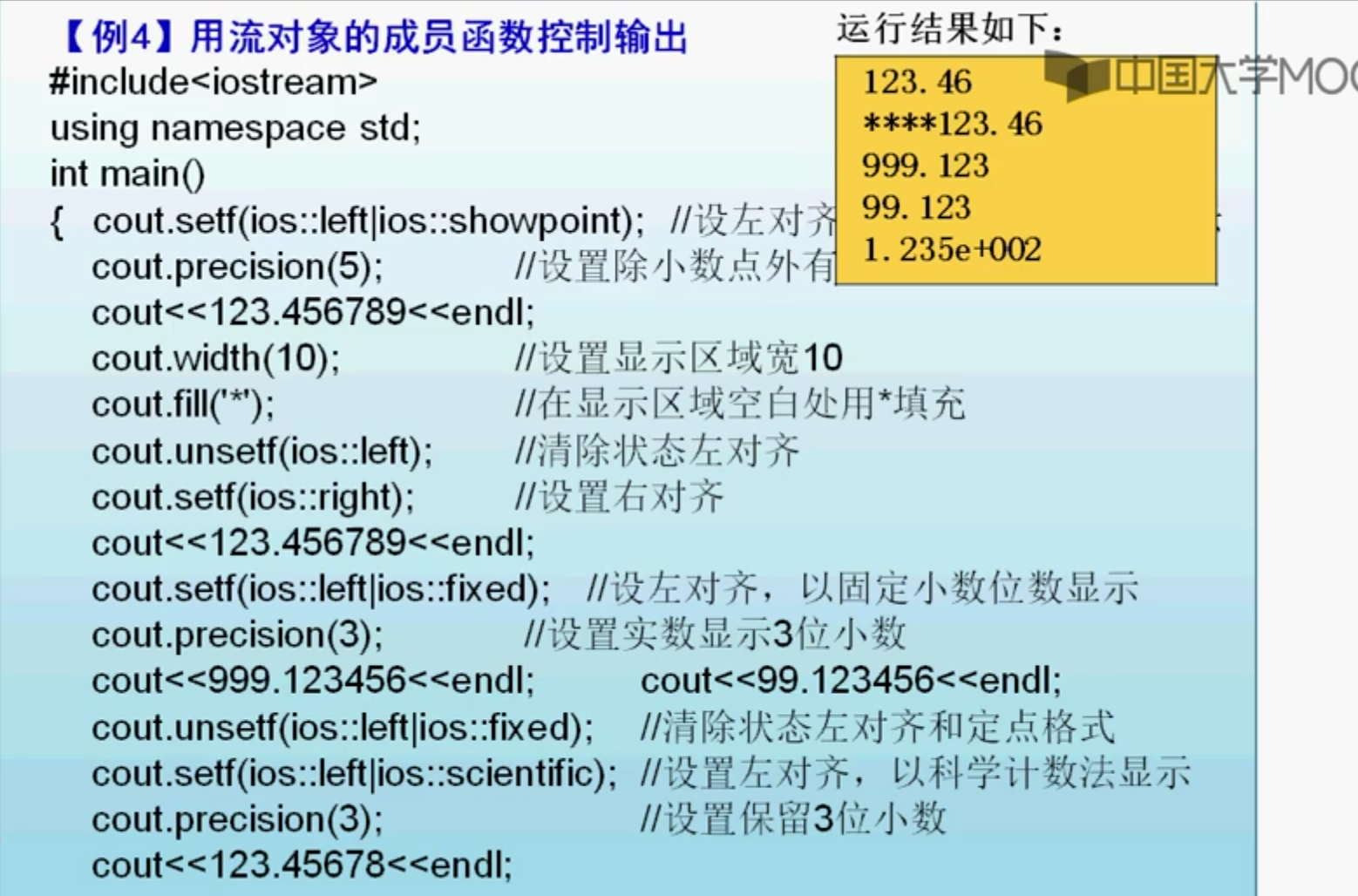3.B 用c++流格式控制符控制输出格式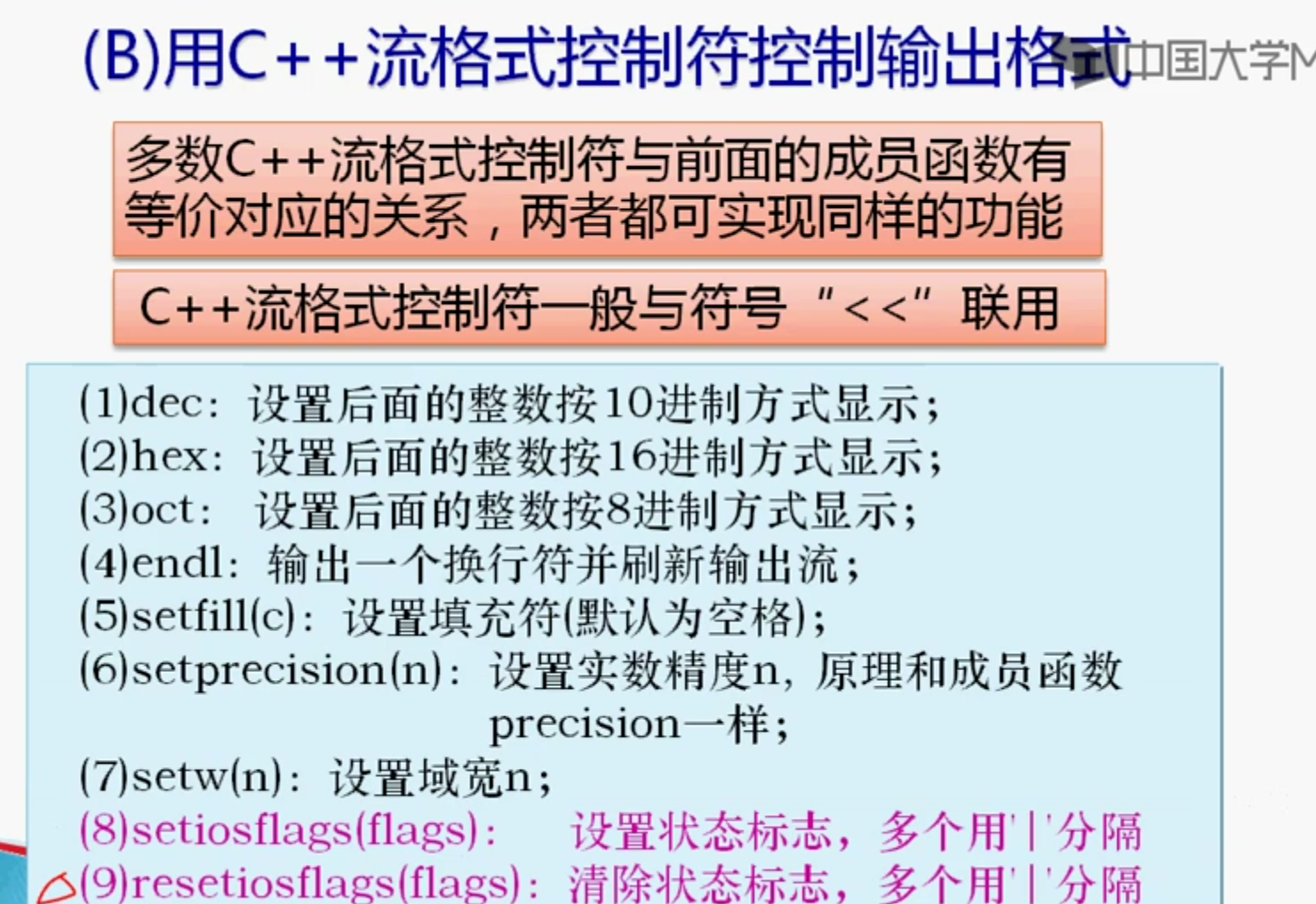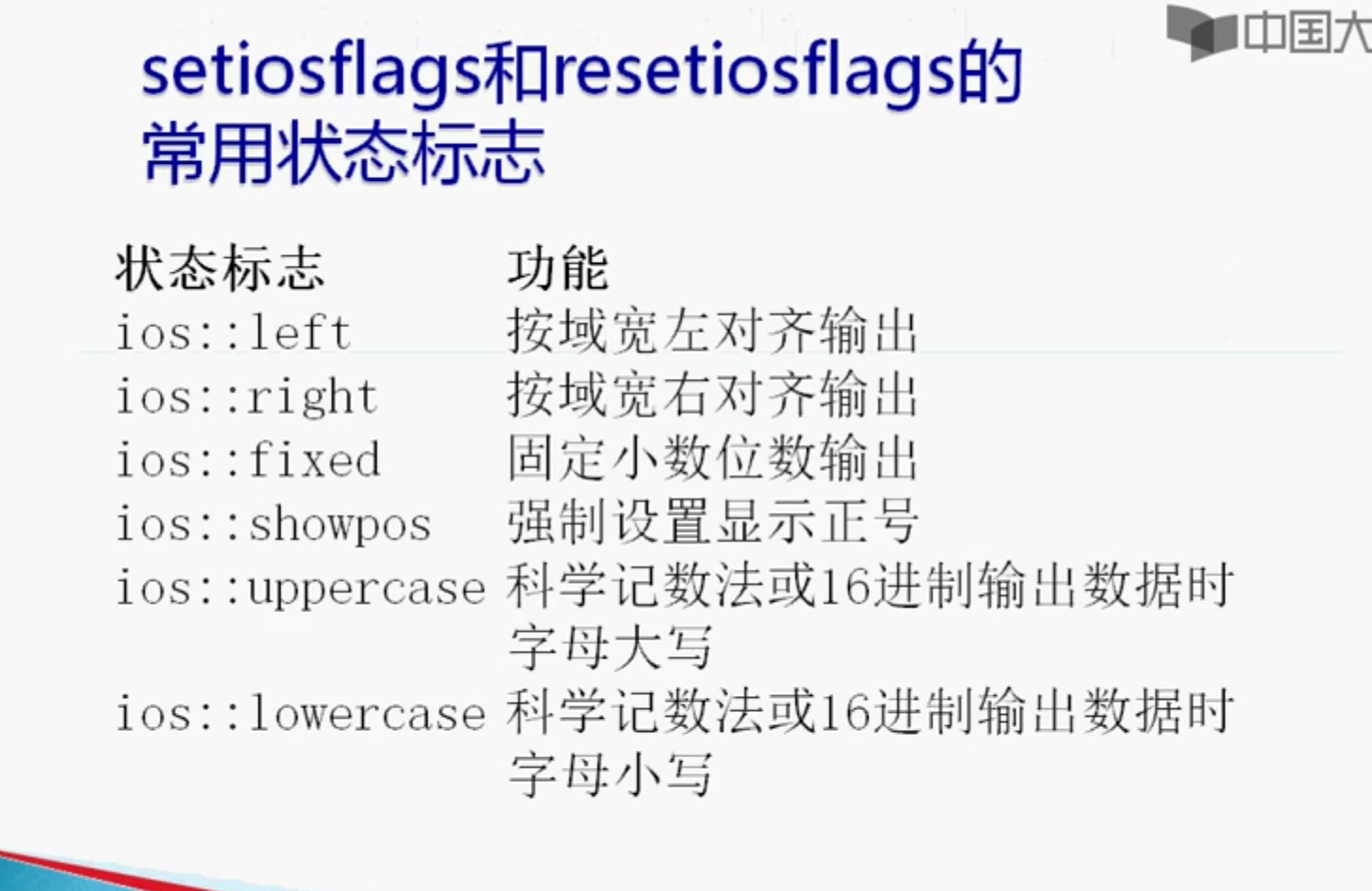#include <iostream>
#include <iomanip>
using namespace std;

int main()
{
int a =128;
cout << dec << a << endl;//10进制
cout << hex << a << endl;
char p[] = "xi'an";
cout << setfill('*') << setw(10) << p << endl;
double b = 12.345;
cout << setiosflags(ios::scientific) << setprecision(8) << b <<  endl;
return 0;
}